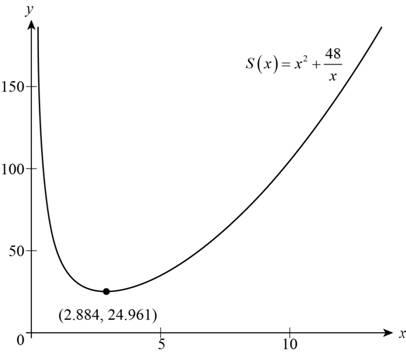# The function that models the surface area of a open box with square base whose volume is 12 ft 3 .### Precalculus: Mathematics for Calcu...

6th Edition
Stewart + 5 others
Publisher: Cengage Learning
ISBN: 9780840068071### Precalculus: Mathematics for Calcu...

6th Edition
Stewart + 5 others
Publisher: Cengage Learning
ISBN: 9780840068071

#### Solutions

Chapter 2, Problem 27P

(a)

To determine

## To find: The function that models the surface area of a open box with square base whose volume is 12 ft3 .

Expert Solution

The function that models the surface area of given box is S(x)=x2+48x .

### Explanation of Solution

Let the dimension of square base be x and height h

Surface area (S) of a open box with square base is,

S=Areaoffoursides+Areaofbottom=4(xh)+(x2) (1)

Volume of the box is,

V=(Areaofbase)(heightofbox)=(x2)(h)=x2h

Substitute 12 for V in above equation.

12=x2h

Divide both sides of above equation by x2 .

12x2=h

Summarize all the information as shown in the table below.

 In Words In Algebra Surface Area 4(x⋅h)+(x2) Length of side of square base. x Height of the box 12x2

Use the information in the table and model the function.

S=4(xh)+(x2)S(x)=4(x(12x2))+(x2)=48x+x2

Thus, the function that models the surface area of given box is S(x)=x2+48x .

(b)

To determine

### To find: The dimensions of the box which minimize the material used to make the box.

Expert Solution

The length of square base of box is approximately 2.88ft and height is 1.44ft .

### Explanation of Solution

The function as calculated in part (a) is,

S(x)=x2+48x

Sketch the graph of above function as shown below.Figure (1)

Observe from the graph shown in Figure (1) that it attains minimum value at x=2.88

Length of square base is 2.88 for minimum surface area.

Height of box as calculated in terms of length of base in part (a) is,

h=12x2

Substitute 2.88 for x in above equation and solve for h.

h=12(2.88)2=128.29=1.44

Thus, the length of square base of box is approximately 2.88ft and height is 1.44ft .

### Have a homework question?

Subscribe to bartleby learn! Ask subject matter experts 30 homework questions each month. Plus, you’ll have access to millions of step-by-step textbook answers!